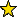# SimplicialDecomposability -- various decomposability routines for simplicial complexes.

## Description

This package includes routines for vertex decomposability and shellability for arbitrary simplicial complexes as well as routines for k-decomposability. Moreover, it can find a shelling order for a shellable simplicial complex.

References:

[BW-1] A. Bjoerner and M. Wachs, "Shellable nonpure complexes and posets, I," Trans. of the AMS 348 (1996), 1299–1327.

[BW-2] A. Bjoerner and M. Wachs, "Shellable nonpure complexes and posets, II," Trans. of the AMS 349 (1997), 3945–3975.

[MT] S. Moriyama and F. Takeuchi, "Incremental construction properties in dimension two: shellability, extendable shellability and vertex decomposability," Discrete Math. 263 (2003), 295–296.

[PB] J. S. Provan and L. J. Billera, "Decompositions of Simplicial Complexes Related to Diameters of Convex Polyhedra," Math. of Operations Research 5 (1980), 576–594.

[St] R. Stanley, "Combinatorics and Commutative Algebra," 2nd edition. Progress in Mathematics, 41. Birkhaeuser Boston, Inc. Boston, MA, 1996.

[Wo] R. Woodroofe, "Chordal and sequentially Cohen-Macaulay clutters," arXiv:0911.4697v1.

## CertificationVersion 1.0.5 of this package was accepted for publication in volume 2 of The Journal of Software for Algebra and Geometry: Macaulay2 on 2010-08-03, in the article Simplicial Decomposability. That version can be obtained from the journal or from the Macaulay2 source code repository.

## Version

This documentation describes version 1.0.6 of SimplicialDecomposability.

## Source code

The source code from which this documentation is derived is in the file SimplicialDecomposability.m2.

## Exports

• Functions and commands
• allFaces -- returns all faces of a simplicial complex, up to a given dimension
• faceDelete -- computes the face deletion for a simplicial complex
• fTriangle -- determines the f-Triangle of a simplicial complex
• hTriangle -- determines the h-Triangle of a simplicial complex
• hVector -- the h-vector of a simplicial complex
• isDecomposable -- determines whether a simplicial complex is k-decomposable
• isSheddingFace -- determines whether a face of a simplicial complex is a shedding face
• isSheddingVertex -- determines whether a vertex of a simplicial complex is a shedding vertex
• isShellable -- determines whether a simplicial complex is shellable
• isShelling -- determines whether a list of faces is a shelling
• isSimplex -- determines whether a simplicial complex is simplex
• isVertexDecomposable -- determines whether a simplicial complex is vertex-decomposable
• shellingOrder -- finds a shelling of a simplicial complex, if one exists
• Methods
• "allFaces(SimplicialComplex)" -- see allFaces -- returns all faces of a simplicial complex, up to a given dimension
• "allFaces(ZZ,SimplicialComplex)" -- see allFaces -- returns all faces of a simplicial complex, up to a given dimension
• "faceDelete(RingElement,SimplicialComplex)" -- see faceDelete -- computes the face deletion for a simplicial complex
• "fTriangle(SimplicialComplex)" -- see fTriangle -- determines the f-Triangle of a simplicial complex
• "hTriangle(SimplicialComplex)" -- see hTriangle -- determines the h-Triangle of a simplicial complex
• "hVector(SimplicialComplex)" -- see hVector -- the h-vector of a simplicial complex
• "isDecomposable(ZZ,SimplicialComplex)" -- see isDecomposable -- determines whether a simplicial complex is k-decomposable
• "isSheddingFace(RingElement,SimplicialComplex)" -- see isSheddingFace -- determines whether a face of a simplicial complex is a shedding face
• "isSheddingVertex(RingElement,SimplicialComplex)" -- see isSheddingVertex -- determines whether a vertex of a simplicial complex is a shedding vertex
• "isShellable(SimplicialComplex)" -- see isShellable -- determines whether a simplicial complex is shellable
• "isShelling(List)" -- see isShelling -- determines whether a list of faces is a shelling
• "isSimplex(SimplicialComplex)" -- see isSimplex -- determines whether a simplicial complex is simplex
• "isVertexDecomposable(SimplicialComplex)" -- see isVertexDecomposable -- determines whether a simplicial complex is vertex-decomposable
• "shellingOrder(SimplicialComplex)" -- see shellingOrder -- finds a shelling of a simplicial complex, if one exists
• Symbols

## For the programmer

The object SimplicialDecomposability is .